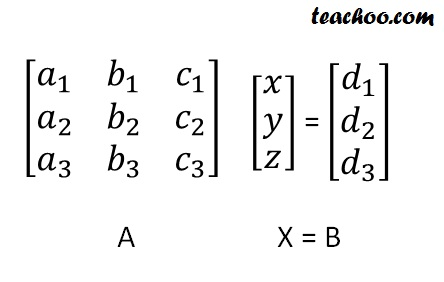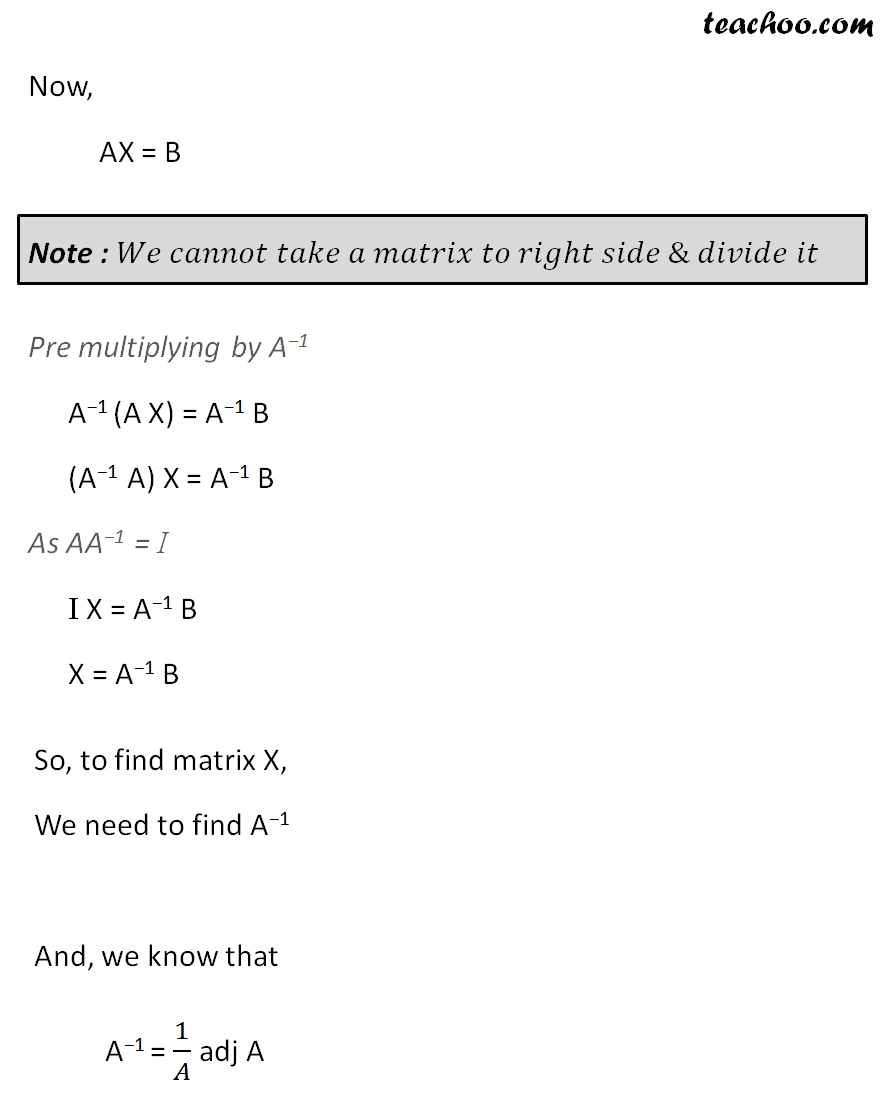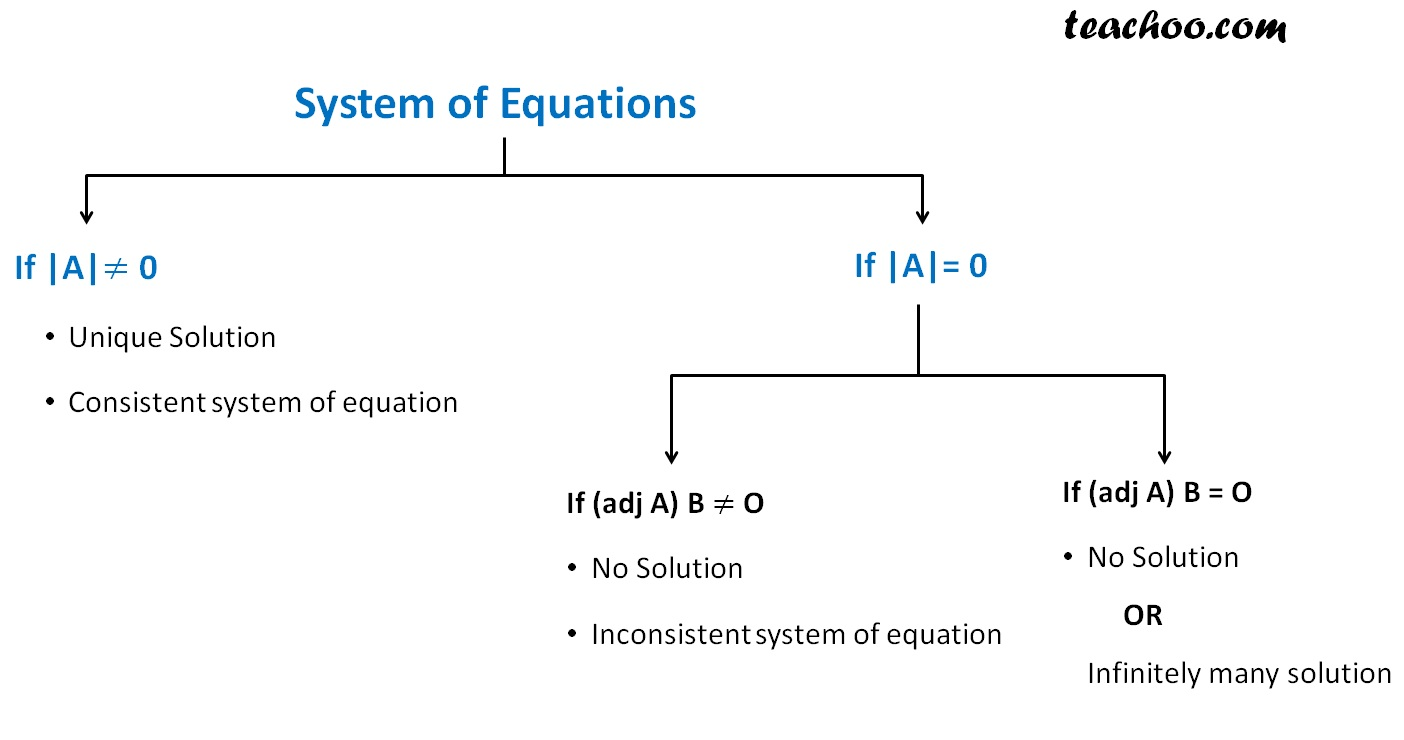Find solution of equations- Equations given

Chapter 4 Class 12 Determinants
Concept wise

Suppose we have to solve

a 1 x + b 1 x + c 1 x = d 1

a 2 x + b 2 x + c 2 x = d 2

a 3 x + b 3 x + c 3 x = d 3

We use matrices to solve this

We write the equation asWe need to find x, y, z

i.e.

We need to find matrix X## But, What if |A| = 0?

If |A| = 0

Then, we calculate (adj A) B

• If (adj A) B ≠ O,
Solution does not exist,
And system of equation is inconsistent

• If (adj A) B = O,
Equation may have infinite solution, or no solution

## To summarize## 3x + 2y = 7

Veiw solution – Example 27

## 4x – 3y + 2z = 4

View solution – Example 28

Learn in your speed, with individual attention - Teachoo Maths 1-on-1 Class